http://cpp.gantep.edu.tr
C++ resources. Faculty of Engineering, University of Gaziantep
MAIN  -  Tutorials  -  Howtos  -  nmPrimer  -  Misc  -  Links  -  Contacts

4. Classes I

Complete: ###################- (95%)

4.1 Introduction
4.2 Structures and classes
4.3 Classes in C++
4.4 Implementation of the Planet Class
4.5 Self Contained Implementation
4.6 Constructors and Destructors
4.7 Pointers to Classes
4.8 Including a Class from a File
4.9 Examples
4.10 Exercises

4.1 Introduction

In the previous topic we saw how user-defined data types are created using the struct keyword. C++ classes (the class) takes this idea further to include member functions and explicit scoping of members and member fucntions.

Although any storage region in RAM is referred to as an object, the word is usually used to describe variables whose data type is a class. Thus object-oriented programming involves programs that use classes.

4.2 Structures and Classes

In its most basic form a class is equivalent to a struct.

For example, the Fruit structure given in the previous topic

```struct Fruit {
double weight;
double price;
};
```
can be rewritten as a class:
```class Fruit {
public:
double weight;
double price;
};
```
The only diffrerenc here is the public keyword.

An example of this basic form of a class is given below where the struct in 03prg01.cpp is rewritten with a class.

04prg01.cpp: Program 03prg01.cpp rewritten as a C++ class.
 ``` 1: 2: 3: 4: 5: 6: 7: 8: 9: 10: 11: 12: 13: 14: 15: 16: 17: 18: 19: 20: 21: 22: 23: 24: 25: 26: 27: 28: 29: 30: ``` ```// Program 03prg01.cpp rewritten as a C++ class. // The only difference is the "public" keyword. #include #include using namespace std; class Fruit{ public: double weight; double price; }; int main(){ Fruit orange, apricot; // prices orange.price = 2.50; // TL/kg apricot.price = 3.25; // TL/kg cout << "Input the amount of orange in kg: "; cin >> orange.weight; cout << "Input the amount of apricot in kg: "; cin >> apricot.weight; cout << "\nTotal prices (TL):\n"; cout << setprecision(2) << fixed; cout << "Orange = " << orange.price * orange.weight << endl; cout << "Apricot = " << apricot.price * apricot.weight << endl; return 0; }``` ```Input the amount of orange in kg: 2 Input the amount of apricot in kg: 1.5 Total prices (TL): Orange = 5.00 Apricot = 4.88 ```

4.3 Classes in C++

The C++ class is a generalization of the struct. Instead of holding only data, a class can hold both data and functions. Note that, in general the use of structures are not recommended in C++ although they are allowed. But you may see some applications.

An object is an instantiation of a class. In terms of variables a class would be the type, and an object would be the variable. Classes are decalred using the class keyword:

```  class class_name {
access_specifier_1:
members_1
access_specifier_2:
members_2
.
.
} object_names;
```

The access specifier is one of the following:

• private
members of a class are accessible only from within other members of the same class.
• public
members are accessible from anywhere where the object is visible.
• protected
members are accessible from members of their same class but also from members of their derived classes.
We will discuss this specifier in the next chapter.

 Note that By default, all members of a class declared with the class keyword have private access for all its members.

For example the following class can be used to represent a planet whose mass is M and radius is R.
```  class Planet{
public:
double Density();
double Gravity();
private:
double M, R, G;
};
```
The functions SetMassRadius(), Density() and Gravity() are called member functions or methods. The variables M, R and G are known as member data.

4.4 Implementation of the Planet Class

 Consider a planet of mass M and equatorial radius R. The mean mass density d and equatorial gravity g of the planet are given respectively by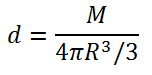and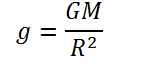where G is the universal gravitational constant and has the value 6.67428x10-11 m3/kg/s. The following example shows how the Planet class could be implemented and used in a C++ program. The data is extracted from NASA: Planets' Physical Characteristics.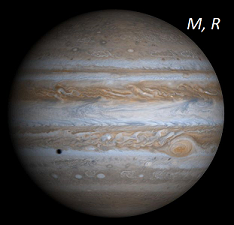04prg02.cpp: A basic use of classes
 ``` 1: 2: 3: 4: 5: 6: 7: 8: 9: 10: 11: 12: 13: 14: 15: 16: 17: 18: 19: 20: 21: 22: 23: 24: 25: 26: 27: 28: 29: 30: 31: 32: 33: 34: 35: 36: 37: 38: 39: ``` ```// A basic use of classes #include #include using namespace std; class Planet{ public: void SetMassRadius(double, double); double Density(); double Gravity(); private: double M, R, G; }; int main(){ Planet Mars; Mars.SetMassRadius(6.4e23, 3.4e6); cout << "Density = " << Mars.Density() << endl; cout << "Gravity = " << Mars.Gravity() << endl; } // Set the mass (kg) and equatorial radius (m) of the planet void Planet::SetMassRadius(double mass, double radius){ M = mass; R = radius; G = 6.67428e-11; } // Mass density in g/cm3 double Planet::Density(){ double d = M/(4.0*M_PI*R*R*R/3); return d * 1.0e-3; } // Surface gravity in m/s2 double Planet::Gravity(){ double g = G*M/(R*R); return g; }``` ```Density = 3.88736 Gravity = 3.6951 ```

Here Mars is declared to be an object of the Planet class. Consequently, Mars has its own internal data members M, R, and G and has also ability call member functions. At line 18, the mass and radius of Mars are supplied via the SetMassRadius() method. Its density and surface gravity are evaluated and output at line 20 and 21. Notice one must use the specifier Planet:: before each member function to indicate that these functions are the members of the Planet class. The output shows that the density of the Mars is about 3.9 g/cm3 and its surface gravity is 3.7 m/s2.

Remember that public members are accessible from outside the class but private members are not. Therefore, the following accesses are forbidden:

```  cout << Mars.M << endl; // forbidden
cout << Mars.R << endl; // forbidden
```

4.5 Self Contained Implementation

Here is the same Planet class with the definitions of its methods included within the class declaration.

04prg03.cpp: Self contained implementation in a class
 ``` 1: 2: 3: 4: 5: 6: 7: 8: 9: 10: 11: 12: 13: 14: 15: 16: 17: 18: 19: 20: 21: 22: 23: 24: 25: 26: ``` ```// Self contained implementation in a class #include #include using namespace std; class Planet{ public: void SetMassRadius(double mass, double radius){ M = mass; R = radius; G = 6.67428e-11; } double Density(){ return 1.0e-3 * M/(4.0*M_PI*R*R*R/3); } double Gravity(){ return G*M/(R*R); } private: double M, R, G; }; int main(){ Planet Mars; Mars.SetMassRadius(6.4e23, 3.4e6); cout << "Density = " << Mars.Density() << endl; cout << "Gravity = " << Mars.Gravity() << endl; }``` ```Density = 3.88736 Gravity = 3.6951 ```

Note that in most cases, the preferred style is to define the member functions outside the class declaration as in the previous program (04prg02.cpp).

4.6 Constructors and Destructors

The Planet class uses the SetMassRadius() function to initialize its objects. However, you can initialize the values when the object is declared like ordinary variables

```  int p = 35;
string name = "Bjarne";
```
This is done by means of a constructor function which is a member function called automatically when an object is declared. A constructor function must have the same name as the class name and have no return type. The following program shows how SetMassRadius() function can be replaced with the constructor.

04prg04.cpp: The use of constructor function
 ``` 1: 2: 3: 4: 5: 6: 7: 8: 9: 10: 11: 12: 13: 14: 15: 16: 17: 18: 19: 20: 21: 22: 23: 24: 25: 26: 27: 28: 29: 30: 31: 32: 33: 34: 35: 36: 37: 38: 39: 40: ``` ```// The use of constructor function #include #include using namespace std; class Planet{ public: Planet(double, double); double Density(); double Gravity(); private: double M, R, G; }; int main(){ Planet Mars(6.4e23, 3.4e6), Jupiter(1.9e27, 7.0e7); cout << "Mars Density = " << Mars.Density() << endl; cout << "Mars Gravity = " << Mars.Gravity() << endl; cout << "Jupiter Density = " << Jupiter.Density() << endl; cout << "Jupiter Gravity = " << Jupiter.Gravity() << endl; } // Constructor function to set the mass and radius of the planet Planet::Planet(double mass, double radius){ M = mass; R = radius; G = 6.67428e-11; } // Mass density in g/cm3 double Planet::Density(){ double d = M/(4.0*M_PI*R*R*R/3); return d * 1.0e-3; } // Surface gravity in m/s2 double Planet::Gravity(){ double g = G*M/(R*R); return g; }``` ```Mars Density = 3.88736 Mars Gravity = 3.6951 Jupiter Density = 1.32242 Jupiter Gravity = 25.8799 ```

Here the declaration at line 16
```  Planet Mars(6.4e23, 3.4e6), Jupiter(1.9e27, 7.0e7);
```
are equivalent to
```  Planet Mars, Jupiter;
```

 Note that One can use default parameter values in the class constructor as follows: ``` // By default, Earth mass and radius are assigned to the planet Planet::Planet(double mass = 6.0e24, double radius = 6.4e6){ M = mass; R = radius; G = 6.67428e-11; } ``` Consider the following declaration ``` Planet p, x(1.0e24, 1e6); ``` Here object p represents the Earth while x represents a different planet.

When an object comes to the end of its life, another member function known as destructor is called automatically. Each class has only one destructor function. Its form for the Planet class can be
```  ~Planet(){
cout << "End of planet\n";
}
```
Example use of the destructor function is illustrated in Section 4.9.

4.7 Pointers to Classes

It is certainly valid to create pointers that point to classes. For example:

```  Planet *pMars;
```
is a pointer to an object of class Planet. In this case, to access directly to a member of an object pointed by a pointer, we can use either the arrow operator (->)
```  pMars->Gravity();
```
or use the statement
```  (*pMars).Gravity();
```
Both notations have the same meaning. Here is a simple example:

04prg05.cpp: A basic use of pointer to a class
 ``` 1: 2: 3: 4: 5: 6: 7: 8: 9: 10: 11: 12: 13: 14: 15: 16: 17: 18: 19: 20: 21: 22: 23: 24: 25: 26: 27: 28: 29: 30: 31: 32: 33: 34: 35: 36: 37: 38: 39: 40: ``` ```// A basic use of pointer to a class #include #include using namespace std; class Planet{ public: Planet(double, double); double Density(); double Gravity(); private: double M, R, G; }; int main(){ Planet *pMars = new Planet(6.4e23, 3.4e6); cout << "Mars Density = " << pMars->Density() << endl; cout << "Mars Gravity = " << pMars->Gravity() << endl; cout << "Mars Density = " << (*pMars).Density() << endl; cout << "Mars Gravity = " << (*pMars).Gravity() << endl; } // Constructor function to set the mass and radius of the planet Planet::Planet(double mass, double radius){ M = mass; R = radius; G = 6.67428e-11; } // Mass density in g/cm3 double Planet::Density(){ double d = M/(4.0*M_PI*R*R*R/3); return d * 1.0e-3; } // Surface gravity in m/s2 double Planet::Gravity(){ double g = G*M/(R*R); return g; }``` ```Mars Density = 3.88736 Mars Gravity = 3.6951 Mars Density = 3.88736 Mars Gravity = 3.6951 ```

Notice you can also use the pointer as follows:
```  Planet Mars(6.4e23, 3.4e6);
Planet *p = &Mars;

cout << "Mars Density = " << p->Density() << endl;
cout << "Mars Gravity = " << p->Gravity() << endl;
```

4.8 Including a Class from a File

The contents of the main program, and of the class(es), can be placed into separate files. Then, using the #include directive you can use the class(es) required. In general, the files containing classes (or functions) are called header files. Usually headers have the extension ".h" or ".hpp".

Consider the declaration of the Planet class and its members are saved in the header file Planet.h as follows:

 ``` 1: 2: 3: 4: 5: 6: 7: 8: 9: 10: 11: 12: 13: 14: 15: 16: 17: 18: 19: 20: 21: 22: 23: 24: 25: 26: 27: 28: 29: 30: 31: 32: 33: 34: 35: 36: 37: ``` ```// Planet.h // Contains the Planet class and its members. #ifndef PLANET_H #define PLANET_H // *** The class declaration *** class Planet{ public: Planet(double, double); double Density(); double Gravity(); private: double M, R, G; }; // *** The member functions *** // Constructor function to set the mass and radius of the planet // By default the planet is assumed to be Earth with M = 6.0e24 kg and R=6400 km. Planet::Planet(double mass = 6.0e24, double radius = 6.4e6){ M = mass; R = radius; G = 6.67428e-11; } // Mass density in g/cm3 double Planet::Density(){ double d = M/(4.0*M_PI*R*R*R/3); return d * 1.0e-3; } // Surface gravity in m/s2 double Planet::Gravity(){ double g = G*M/(R*R); return g; } #endif```

Here at line 4, #ifndef (if not defined) checks whether the symbolic constant PLANET_H has been #defined earlier. If it has been already defined before the declarations and members of the Planet class will not be included to your program. This operation prevents the repeated declaration of the Planet class.

Now, you can include this header file in your main program given below (assuming that Planet.h and 04prg06.cpp are in the same folder).

04prg06.cpp: Including a class from a file
 ``` 1: 2: 3: 4: 5: 6: 7: 8: 9: 10: 11: 12: 13: 14: 15: 16: ``` ```// Including a class from a file #include #include using namespace std; #include "Planet.h" int main(){ Planet Mars(6.4e23, 3.4e6), Jupiter(1.9e27, 7.0e7); cout << "Mars Density = " << Mars.Density() << endl; cout << "Mars Gravity = " << Mars.Gravity() << endl; cout << "Jupiter Density = " << Jupiter.Density() << endl; cout << "Jupiter Gravity = " << Jupiter.Gravity() << endl; }``` ```Mars Density = 3.88736 Mars Gravity = 3.6951 Jupiter Density = 1.32242 Jupiter Gravity = 25.8799 ```

4.9 Examples

EXAMPLE 1 A Cat class: Each object of this class will represent a cat. The class includes* a constructor function whose prototype is Cat(int Age=1, double Mass=2.0); to set (initialize) the age and weight of the cat. * a member function named void speak() that outputs a "meow" message. * a member function named void kill() that reduces the cat's lives by one (the cat has nine lives). * a member function named double getMass() to get the mass of the cat. * a member function named int getAge() to get the age of the cat. * a member function named int getLife() to get the remaining life(s) of the cat. Have fun.

04prg07.cpp: A Cat class implementation
 ``` 1: 2: 3: 4: 5: 6: 7: 8: 9: 10: 11: 12: 13: 14: 15: 16: 17: 18: 19: 20: 21: 22: 23: 24: 25: 26: 27: 28: 29: 30: 31: 32: 33: 34: 35: 36: 37: 38: 39: 40: 41: 42: 43: 44: 45: 46: 47: 48: 49: 50: ``` ```// A Cat class implementation #include // The Cat class declaration class Cat{ private: double m_mass; int m_age, m_life; public: Cat(int = 1, double =2.0); ~Cat(); void speak(); void kill(); double getMass(){ return m_mass; } int getAge() { return m_age; } int getLife(){ return m_life; } }; int main(){ Cat Tekir(1, 3.0); Tekir.speak(); std::cout << "The Age = " << Tekir.getAge() << std::endl; std::cout << "The Mass = " << Tekir.getMass() << std::endl; std::cout << "The Life = " << Tekir.getLife() << std::endl; Tekir.kill(); std::cout << "The Life = " << Tekir.getLife() << std::endl; } // constructor function Cat::Cat(int Age, double Mass){ m_age = Age; m_mass = Mass; m_life = 9; std::cout << "--- Start of the Cat class ---\n"; } // Destructor do nothing Cat::~Cat(){ std::cout << "--- End of the Cat class ---\n"; } // speak method void Cat::speak(){ std::cout << "meow..\a\n"; } // kill method to kill the cat by one void Cat::kill(){ m_life--; if(m_life<0) { m_life = 0; std::cout << "Cat has already died.\n"; } }``` ```--- Start of the Cat class --- meow.. The Age = 1 The Mass = 3 The Life = 9 The Life = 8 --- End of the Cat class --- ```

This class has five member functions; speak() and kill() are declared outside the class while getMass(), getAge() and getLife() are self contained. Note that, traditionally, each private data member starts with m_ symbol.

EXAMPLE 2 A Vector class: Each object of this class will represent a vector in the x-y plane. The class includes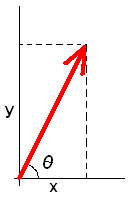* a constructor function whose prototype is Vector(double x=0, double y=0); to set (initialize) the components. * a member function named double magnitude() that returns the norm of the vector. * a member function named double theta() that returns the angle in degrees between the vector direction and x-axis. * a member function named void scale(double c) to scale the vector by a constant c. * a member function named void unitvector(double &ux, double &uy) to set the components the of the unit vector in the direction of the vector.

04prg08.cpp: A Vector class implementation
 ``` 1: 2: 3: 4: 5: 6: 7: 8: 9: 10: 11: 12: 13: 14: 15: 16: 17: 18: 19: 20: 21: 22: 23: 24: 25: 26: 27: 28: 29: 30: 31: 32: 33: 34: 35: 36: 37: 38: 39: 40: 41: 42: 43: 44: 45: 46: 47: 48: 49: 50: 51: ``` ```// A Vector class implementation #include #include using namespace std; // The Vector class declaration class Vector{ private: double m_x, m_y; public: Vector(double =0.0, double =0.0); double magnitude(); double theta(); void scale(double); void unitvector(double &, double &); }; int main(){ Vector v(3.0, 4.0); double ux, uy; cout << v.magnitude() << endl; v.scale(2); cout << v.magnitude() << endl; cout << v.theta() << endl; v.unitvector(ux, uy); cout << ux << '\t' << uy << endl; } // Constructor function Vector::Vector(double x, double y){ m_x=x; m_y=y; } // Magnitude (norm) of the vector double Vector::magnitude(){ return sqrt(m_x*m_x + m_y*m_y); } // Angle in degrees between the vector direction and x-axis double Vector::theta(){ return atan(m_y/m_x) * 180.0/M_PI; } // Scale the vector by a constant c void Vector::scale(double c){ m_x *= c; m_y *= c; } // Components the of the unit vector in the direction of the vector void Vector::unitvector(double &ux, double &uy){ ux = m_x/magnitude(); uy = m_y/magnitude(); }``` ```5 10 53.1301 0.6 0.8 ```

4.10 Exercises

1. What is the main difference between a structure and a class in C++?
2. What is the action of the public in a class?
3. What is the action of the private in a class?
4. What name must a constructor have?
5. What name must a destructor have?

6. Write down the output of the following program:
```#include <iostream>

class Particle{
private:
double v0, x0, a, m;
public:
Particle(double mass){
v0 = x0 = a = 0.0; m = mass;
}
void SetInitialValues(double pos, double vel, double acc){
v0 = vel; x0 = pos;  a  = acc;
}
double GetPosition(double t){ return x0 + v0*t + 0.5*a*t*t; }
double GetVelocity(double t){ return v0+a*t; }
double GetKineticEnergy(double t){
return 0.5*m*GetVelocity(t)*GetVelocity(t);
}
};

int main(){
Particle *ball = new Particle(2.0);
double time = 0.0;

ball->SetInitialValues(0.1, 12.0, -9.8);

while(time<=2.0){
std::cout << time << '\t'
<< ball->GetPosition(time)  << '\t'
<< ball->GetVelocity(time)  << '\t'
<< ball->GetKineticEnergy(time) << std::endl;
time += 0.5;
}
}
```
 5.   In the x-y plane, the general equation of a circle of radius r is given by: (x - a)2 + (y - b)2 = r2. Implement a Circle class. Each object of this class will represent a circle, storing its radius (r) and the a and b coordinates of its center as doubles. The class must include a default constructor function whose prototype is Circle(double radius, double centerX, double centerY); to set (initialize) radius and center coordinates. a member function named double area() that returns the area of the circle. a member function named double circ() that returns circumference. a member function named bool isInside(double x, double y) that returns true if the given point (x, y) is inside the circle and returns false otherwise. Assume that the class decleration and its members/methods are stored in the file Circle.h. An example usage of the Circle is given below: ```#include using namespace std; #include "Circle.h" int main(){ // a circle whose center is origin Circle guzelCember(10.0, 0.0, 0.0); cout << guzelCember.area() << endl; cout << guzelCember.circ() << endl; cout << guzelCember.isInside(1.5, 2.7) << endl; return 0; } ```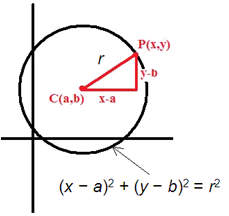6.   Implement an RCcircuit class. Each object of this class will represent a simple charging RC circuit. The class must include a default constructor function whose prototype is RCcircuit(double R, double C, double V0); to initialize the values of resistance (R) in Ohms, capacitor (C) in Farads and the potential difference across DC voltage source (V0) in Volts. a member function named double current(double t) that returns the current in the circuit at given time (in seconds) where t > 0. a member function named double VC(double t) that returns potential across the capacitor at given time (in seconds) where t > 0 . a member function named double VR(double t) that potential across the capacitor at given time (in seconds) where t > 0. a member function named double tau() that returns the time constant of the circuit defined by T = R*C. Assume that the class decleration and its members/methods are stored in the file RCCircuit.h. Example usage of the RCCircuit class is given below: ```#include using namespace std; #include "RCCircuit.h" int main(){ RCcircuit *Devrem = new RCcircuit(2.2e+6, 1.0e-6, 12.); double time = 0.0; cout << "time constant: " << Devrem->tau() << endl; do{ cout << Devrem->current(time) << "\t" << Devrem->VC(time) << "\t" << Devrem->VR(time) << endl; time += 0.1; }while(time < 5*Devrem->tau()); return 0; } ```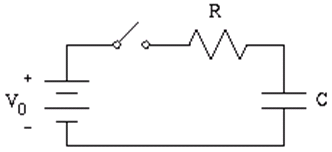[index][<][>]